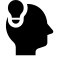# LeetCode 969 Pancake Sorting

## Solution of C++

Posted by viewsetting on May 17, 2019

# Problem

Given an array A, we can perform a pancake flip: We choose some positive integer k <= A.length, then reverse the order of the first k elements of A. We want to perform zero or more pancake flips (doing them one after another in succession) to sort the array A.

Return the k-values corresponding to a sequence of pancake flips that sort A. Any valid answer that sorts the array within 10 * A.length flips will be judged as correct.

Note:

1. 1 <= A.length <= 100
2. A[i] is a permutation of [1, 2, ..., A.length]

# Example

### 1

Input: [3,2,4,1]
Output: [4,2,4,3]
Explanation:
We perform 4 pancake flips, with k values 4, 2, 4, and 3.
Starting state: A = [3, 2, 4, 1]
After 1st flip (k=4): A = [1, 4, 2, 3]
After 2nd flip (k=2): A = [4, 1, 2, 3]
After 3rd flip (k=4): A = [3, 2, 1, 4]
After 4th flip (k=3): A = [1, 2, 3, 4], which is sorted.


### 2

Input: [1,2,3]
Output: []
Explanation: The input is already sorted, so there is no need to flip anything.
Note that other answers, such as [3, 3], would also be accepted.


# Solution

The given sequence is not sorted, if we want to shrink the size of the state, we can arrange the

# Code

class Solution {
public:
bool vis;
int find_idx(int val,vector<int>& tem,int len)
{

for(int i=0;i<len;i++)
{
if(tem[i]==val)
{
//vis[i]=1;
return i;
}
}
return -1;
}
void rev(vector<int>& tem,int k)
{
for(int i=0;i<(k+1)/2;++i)
{
swap(tem[i],tem[k-i]);
//vis[i]=vis[k-i-1];
}
}
vector<int> pancakeSort(vector<int>& A) {
memset(vis,0,sizeof(vis));
vector<int> res;
auto cpy = A;
sort(cpy.begin(),cpy.end());
int N = A.size();
for(int i=N-1;i>=0;--i)
{
if(A[i]==cpy[i]) continue;
int idx=find_idx(cpy[i],A,i+1);
if(idx+1!=1)
res.push_back(idx+1);
rev(A,idx);
if(i+1!=1)
res.push_back(i+1);
rev(A,i);
}
return res;
}
};